# Test: Monotonicity (Competition Level) - 2

## 16 Questions MCQ Test Mathematics (Maths) Class 12 | Test: Monotonicity (Competition Level) - 2

Description
This mock test of Test: Monotonicity (Competition Level) - 2 for JEE helps you for every JEE entrance exam. This contains 16 Multiple Choice Questions for JEE Test: Monotonicity (Competition Level) - 2 (mcq) to study with solutions a complete question bank. The solved questions answers in this Test: Monotonicity (Competition Level) - 2 quiz give you a good mix of easy questions and tough questions. JEE students definitely take this Test: Monotonicity (Competition Level) - 2 exercise for a better result in the exam. You can find other Test: Monotonicity (Competition Level) - 2 extra questions, long questions & short questions for JEE on EduRev as well by searching above.
QUESTION: 1

Solution:
QUESTION: 2

Solution:
QUESTION: 3

### The function f(x) = x(x + 3) e–x/2 satisfies all the conditions of Rolle's theorem in [–3, 0]. The value of c which verifies Rolle's theorem, is

Solution:
QUESTION: 4

For what values of a does the curve

f(x) = x(a2 – 2a – 2) + cos x is always strictly monotonic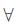x ∈ R.

Solution:
QUESTION: 5

If the function f(x) = x3 – 6ax2 + 5x satisfies the conditions of Lagrange's mean theorem for the interval [1, 2] and the tangent to the curve y = f(x) at x = 7/4 is parallel to the chord joining the points of intersection of the curve with the ordinates x = 1 and x = 2. Then the value of a is

Solution:
QUESTION: 6

The function f(x) = tan-1 (sin x + cos x) is an increasing function in

Solution:
QUESTION: 7

A function y = f(x) has a second order derivative f" = 6(x – 1). If its graph passes through the point (2, 1) and at that point the tangent of the graph is y = 3x – 5, then the function is

Solution:
QUESTION: 8

If f(x) = [a sin x + b cosx] / [c sin x + d cos x] is monotonically increasing, then

Solution:
QUESTION: 9

The equation xex = 2 has

Solution:
QUESTION: 10

If f(x) = 1 + x l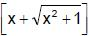and g(x) =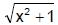then for x ³ 0

Solution:
QUESTION: 11

The set of values of the parameter `a' for which the function; f(x) = 8ax – a sin 6x – 7x – sin 5x increases & has no critical points for all x Î R, is

Solution:
QUESTION: 12

f : [0, 4] → R is a differentiable function then for some a, b Î (0, 4), f2(4) – f2(0) equals

Solution:
QUESTION: 13

If 0 < a < b <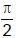and f(a, b) =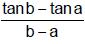, then

Solution:
QUESTION: 14

Let f(x) = ax4 + bx3 + x2 + x – 1. If 9b2 < 24a, then number of real roots of f(x) = 0 are

Solution:
QUESTION: 15

If f(x) = (x – 1) (x – 2) (x – 3) (x – 4), then roots of f'(x) = 0 not lying in the interval

Solution:
QUESTION: 16

If f(x) = 1 + xm (x – 1)n, m, n ∈ N, then f'(x) = 0 has atleast one root in the interval

Solution: